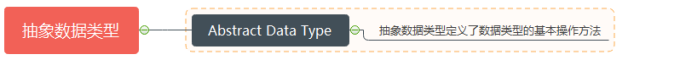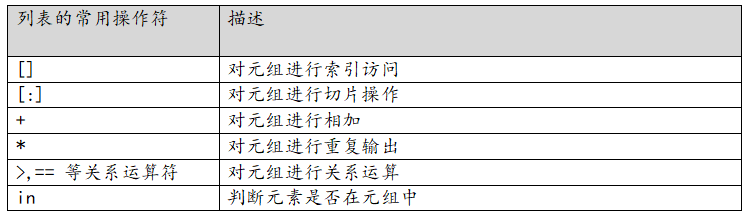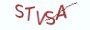Python基础教程

# 11.2.1 元组的常用操作符# 11.2.2 []操作符: 索引访问元组

`element = tuple[index]`

Python中的索引分为正索引和负索引。正索引从0开始进行编号，表示数据集合中的第一个元素。负索引从-1开始编号，表示数据集合中的倒数第一个元素。正负索引值必须在有效的范围之内，否则会抛出越界访问的错误信息。对此不熟悉的同学，可以复习9.2节中的内容。

```# __desc__ = 通过索引来访问元组中的元素

numbers = (1,2,3,4)

# 1.读取元组中的第一个元素
first_number = numbers

# 2.读取元组中的最后一个元素
last_number = numbers[-1]```

# 11.2.3  [:]操作符：元组的切片

`new_tuple = tuple[:]`

```# __desc__ = 对元组执行切片操作

# 定义元组类型变量
numbers = (1,2,3,4)

container = numbers[0:2]
# container的输出为(1,2)

container = numbers[:2]
# container的输出为(1,2)

container = numbers[2:]
# container的输出为(3,4)

container = numbers[-3:-1]
# container的输出为(2,3)```

# 11.2.4 +操作符：元组的加法

`new_tuple = tuple0 + tuple1`

```# __desc__ = 对元组执行加法操作

# 定义变量negative_numbers表示负数
negative_numbers = (-1,-2,-3,-4)

# 定义变量 positive_numbers表示正数
positive_numbers = (1,2,3,4)

# 定义变量 natural_numbers表示自然数
natural_numbers =  negative_numbers + positive_numbers
# natural_numbers的输出为(-1,-2,-3,-4,1,2,3,4)```

# 11.2.5  *操作符：元组的乘法

"*" 操作符用来对元组进行重复输出，返回一个新的列表。

`new_tuple = tuple * number`

```# __desc__ = 对元组执行乘法操作

numbers = (-1,-2,-3)
new_numbers = numbers * 2

# new_numbers的输出为(-1,-2,-3, -1,-2,-3)

# *号后的整型值小于等于0时，输出空元组
empty = numbers * 0
# empty为空元组```

# 11.2.6 元组的关系运算

`boolean = tuple0 > tuple1`

```# __desc__ = 对元组执行关系运算

left = (1,3,99)
right = (2)

result = left > right
# result的输出为False```

```# __desc__ = 通过while循环来判断元组是否相等

left = (3,3,3,3,99,3)
right = (3,3,3,3,3,109)

# 执行全局的len方法来获取复合数据类型的元素数
length_of_left = len(left)
length_of_right = len(right)

length = length_of_left if length_of_left < length_of_right else length_of_right
index = 0

'''

'''
is_equal = True

# 以最小的元组长度来进行遍历
# 这样可以避免访问越界
while index < length:
if left[index] != right[index]:
is_equal = False
break
elif left[index] < right[index]:
is_equal = False
break
index += 1
else:
# 如果正常退出while循环，说明在最小长度内，元组的元素都相等
is_equal = False if length_of_left != length_of_right else True```

# 11.2.7  in操作符：查找元素

`boolean = element in tuple`

`boolean = element not in tuple`

```# __desc__ = 通过in操作符来执行元素查找

natural_numbers = (1,2,3,4,5,6,7,8,9)
number = 99

if number in natural_numbers:
# 如果number存在与列表中，那么表达的输出为True
print("{} in numbers:{}".format(number, numbers))
else:
print("there is no {} in numbers {}".format(number, numbers))```

# 11.2.8 知识要点

(1)元组类型是一种线性的序列结构，可以通过索引来访问元组中的元素。
(2)元组与字符串都是一种静态的数据类型，只能通过索引来读取字符，不能对字符进行修改。列表类型可以通过索引来对元素进行修改。
(3)元组的关系运算是按序进行比较的，这里的按序比较是从元组的第一个元素开始，逐元素进行比较。只有在元素值相同，顺序也相同的情况下，两个元组才相等，否则以元素先后的大小关系来确定元组的大小关系。

# 11.2.9 最具实力的小班培训

`(1) Python后端工程师高薪就业班，月薪11K-18K，免费领取课程大纲(2) Python爬虫工程师高薪就业班，年薪十五万，免费领取课程大纲(3) Java后端开发工程师高薪就业班，月薪11K-20K, 免费领取课程大纲(4) Python大数据分析，量化投资就业班，月薪12K-25K,免费领取课程大纲`### 已有4位薯条发表了看法：

•访客  评论于 2020-06-02 10:51:45  回复

谢谢老师的解读，通途易懂

•薯条老师  评论于 2020-06-02 16:17:26  回复

不客气

•访客  评论于 2020-12-23 16:50:07  回复

11.2.7 in操作符：查找元素
format参数错误，第二个参数应该为natural_numbers

•访客  评论于 2020-12-23 16:50:18  回复

11.2.7 in操作符：查找元素
format参数错误，第二个参数应该为natural_numbers

## 注册账号